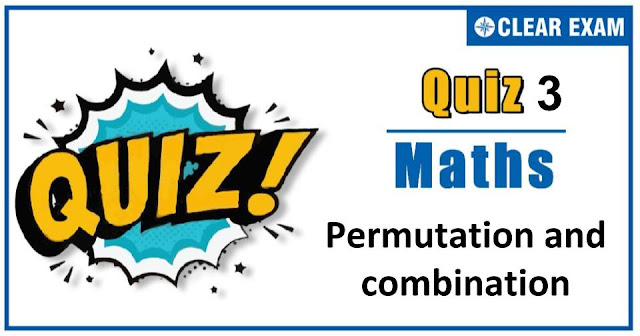## [LATEST]\$type=sticky\$show=home\$rm=0\$va=0\$count=4\$va=0

As per analysis for previous years, it has been observed that students preparing for JEE MAINS find Mathematics out of all the sections to be complex to handle and the majority of them are not able to comprehend the reason behind it. This problem arises especially because these aspirants appearing for the examination are more inclined to have a keen interest in Mathematics due to their ENGINEERING background.

Furthermore, sections such as Mathematics are dominantly based on theories, laws, numerical in comparison to a section of Engineering which is more of fact-based, Physics, and includes substantial explanations. By using the table given below, you easily and directly access to the topics and respective links of MCQs. Moreover, to make learning smooth and efficient, all the questions come with their supportive solutions to make utilization of time even more productive. Students will be covered for all their studies as the topics are available from basics to even the most advanced.

Q1. There are n-points in a plane of which p points are collinear. How many lines can be formed from these points?
•  nC2-pC2
•  n-nC2
•  nC2-nP2-1
•  nC2-nP2+1
Solution
(d) nC2-nP2+1

Q2.The number of divisors of the number of 38808 (excluding 1 and the number itself)is
•  72
•  70
•  71
•  None of these
Solution
(b) Since, 38808=23×32×72×111 ∴ Number of divisors =4×3×3×2-2 =72-2=70

Q3.  The number of ways in which 5 boys and 5 girls can be seated for a photograph so that no two girls sit next to each other is
•   10!/(5!2)
•  10!/((5!))
•  (5!)2
•  6!.5!
Solution
(d) Required number of ways=5!×6!

Q4. All possible two-factor products are formed from the no numbers 1,2,…,100. The number of factors out of the total obtained which are multiple of 3 is
•  2211
•  4950
•  None of these
•  2739
Solution
(d) The total number of two factor products = 100C2. Out of the numbers 1,2,3,…,100; the multiples of 3 are 3,6,9,…,99 i.e., there are 33 multiples of 3, and therefore there are 67 non-multiples of 3 So, the number of two factor products which are not multiples of 3= 67C2 So, the required number = 100C2-67C2 =2739

Q5. Ten different letters of an alphabet are given. Words with five letters are formed from these given letters. Then the number of words which have at least one letter repeated, is
•  69760
•  30240
•  99748
•  None of these
Solution
(a) The total number of ways in which words with five letters are formed from given 10 letters =105=100000 Total number of ways in which words with five letters are formed (no repetition) =10×9×8×7×6=30240 ∴ Required number of ways =100000-30240=69760

Q6. The number of words which can be made out of the letters of the word “MOBILE” when consonants always occupy odd places, is
•  20
•  36
• 30
•  720
Solution
(b) There are 6 letters in the word ‘MOBILE’. Consequently, there are 3 odd places and 3 even places. Three consonants M,B and L can occupy three odd places in 3 ! ways. Remaining three places can be filled by 3 vowels in 3 ! ways. Hence, required number of words =3 !×3 !=36

Q7. The number of triangles that can be formed by 5 points in a line and 3 points on a parallel line is
•  8C3
•  8C3-5C3
•  8C3-5C3-1
•  None of these
Solution
(c) 8C3-5C3-1

Q8. If a polygon has 44 diagonals, then the number of its sides are
•  7
•  11
•  8
•  None of these
Solution
(b) Let n be the number of diagonals of a polygon. Then, nC2-n=44 ⇒(n(n-1))/2-n=44 ⇒n2-3n-88=0 ⇒n=-8 or 11 ∴n=11

Q9. If eight persons are to address a meeting, then the number of ways in which a specified speaker is to speak before another specified speaker is
•  2520
•  40320
•  None of these
•  20160
Solution
(d) Let A,B be the corresponding speakers. Without any restriction the eight persons can be arranged among themselves in 8 ! ways; but the number of ways in which B speaks A speaks before B and the number of ways in which B speaks before A make up 8 !. Also, the number of ways in which A speaks before B is exactly same as the number of ways in which B speaks before A. So, the required number of ways =1/2 (8 !)=20160

Q10. The sum of all that can be formed with the digits 2,3,4,5 taken all at a time is
•  84844
•  93324
•  66666
• None of these
Solution
(b) We have, Required sum =(2+3+4+5)(4-1) !((104-1)/(10-1)) =14×6×((104-1)/(10-1))=93324## Want to know more

Want to Know More
Please fill in the details below:

## Latest NEET Articles\$type=three\$c=3\$author=hide\$comment=hide\$rm=hide\$date=hide\$snippet=hide

Name

ltr
item
Best IIT JEE Coaching Institute in Delhi | NEET Coaching Institute in Delhi: Permutation-and-combination-quiz-3
Permutation-and-combination-quiz-3
https://1.bp.blogspot.com/-UlXOMJCXnmk/X3iteJlwIlI/AAAAAAAAAYw/NR002LtbApMLoqyfGKMBbWhHDACxu_axQCLcBGAsYHQ/w640-h336/Quiz%2BImage%2BTemplate%2B%25282%2529.jpg
https://1.bp.blogspot.com/-UlXOMJCXnmk/X3iteJlwIlI/AAAAAAAAAYw/NR002LtbApMLoqyfGKMBbWhHDACxu_axQCLcBGAsYHQ/s72-w640-c-h336/Quiz%2BImage%2BTemplate%2B%25282%2529.jpg
Best IIT JEE Coaching Institute in Delhi | NEET Coaching Institute in Delhi
https://www.cleariitmedical.com/2020/10/permutation-and-combination-quiz-3.html
https://www.cleariitmedical.com/
https://www.cleariitmedical.com/
https://www.cleariitmedical.com/2020/10/permutation-and-combination-quiz-3.html
true
7783647550433378923
UTF-8